# Procedure for finding local and endpoint extreme values using the higher derivative test

(diff) ← Older revision | Latest revision (diff) | Newer revision → (diff)

This article describes the procedure for finding the local and endpoint extreme values for a twice differentiable function using the second derivative test, where, in cases that the test is inconclusive, we switch to the higher derivative test.

## Procedure for twice differentiable function on an interval

Suppose$f$ is a continuous function on an interval (may be open, closed, half-closed, or infinite in one or both directions). Further, suppose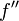$f''$ is defined everywhere on the interior of the interval. Then the procedure is as follows:

1. Compute the formal expressions for the first derivative$f'$ and the second derivative$f''$ (note that for the method to apply, both these must be defined everywhere on the interior of the interval).
2. Find all the points where$f'$ takes the value zero. These are precisely the critical points for$f$.
3. Evaluate$f''$ at each of these critical points and determine the sign of$f''$ on each critical point. Then, by the second derivative test:
• Any critical point where$f''$ takes a negative value is a point of local maximum for$f$.
• Any critical point where$f''$ takes a positive value is a point of local minimum for$f$.
• For a critical point where$f''$ takes the value zero, the second derivative test is inconclusive, i.e., the point may be a point of local maximum, local minimum, or neither -- we don't know yet.
4. If there are points where the second derivative test is inconclusive, compute the formal expression for the third derivative. Evaluate the third derivative at all the points where the second derivative test has been inconclusive. For those points where the third derivative is nonzero, we can conclude that the point is neither a point of local maximum nor a point of local minimum. For those points where the third derivative evaluates to zero, the test is still inconclusive. If there are such points, we pass to the next derivative.
5. The overall procedure is as follows: We keep formally computing more derivatives until all the critical points have some derivative evaluated to a nonzero value. If the first nonzero value occurs at an odd derivative, then the point is not a point of local max/min. If the first nonzero value occurs at an even derivative, then the point is a point of local max/min -- max if the value is negative, and min if the value is positive.
6. For the endpoints: If the one-sided derivative exists and is nonzero at an endpoint, we can simply use the sign of that to determine the nature of the endpoint extreme value. If the one-sided derivative value is zero, we can use the one-sided version of second derivative test at the endpoint, and if that fails, then the one-sided version of higher derivative test.

Note that this test may run into inconclusive situations. For instance, it may be the case that higher derivatives cease to exist before becoming nonzero. It may also be the case that all the higher derivative values are zero, in which case the test is again inconclusive. However, the higher derivative test does settle a lot of situations that are left ambiguous by the second derivative test.

### Case of function with periodic derivative and infinitely many critical points

If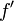$\! f'$ is a periodic function, say with period$h$, then its set of critical points is invariant under translation by$h$. This means that if$c$ is a critical point, so is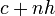$c + nh$ for any$n \in \mathbb{Z}$.

In this case, it is obviously not feasible to do a sign analysis of$f''$ separately for any critical point. However, the good news is that we do not need to. It suffices to do the sign analysis of values of$f''$ at critical ponits over a single period and then extrapolate to the entire domain.

Note that this remark applies even if$f$ itself is not a periodic function. For instance, it applies to the study of functions of the form (linear) + (trigonometric), such as$x \mapsto x - 2 \sin x$.

### Example of a polynomial function

Consider the function:$f(x) := 3x^5 - 5x^3 + 7, \qquad x \in [-2,3]$

1. Computation of$f'$ and$f''$:$f'(x) = 15x^4 - 15x^2$ and$f''(x) = 60x^3 - 30x$.
2. Points where$f'$ takes the value 0: We have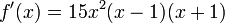$f'(x) = 15x^2(x - 1)(x + 1)$. The roots are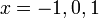$x = -1,0,1$. These are all in the interval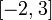$[-2,3]$, hence are precisely the critical points of$f$.
3. Evaluate the sign of$f''$ at each critical point:
• At the critical point$-1$: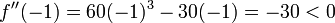$f''(-1) = 60(-1)^3 - 30(-1) = -30 < 0$, so -1 is a point of local maximum for$f$.
• At the critical point$0$: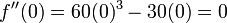$f''(0) = 60(0)^3 - 30(0) = 0$, so the second derivative test is inconclusive at 0.
• At the critical point$1$:$f''(1) = 60(1)^3 - 30(1) = 30 > 0$, so 1 is a point of local minimum for$f$.
4. We notice that there is a critical point, namely$x = 0$, for which the second derivative test has been inconclusive. We thus compute the third derivative formally and evaluate at this point. The third derivative is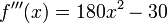$f'''(x) = 180x^2 - 30$. Evaluation at 0 gives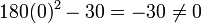$180(0)^2 - 30 = -30 \ne 0$. Since this is nonzero, we conclude that$x = 0$ is not a point of local extremum.
5. (The next step, involving higher derivatives, is unnecessary).
6. For the endpoints: The one-sided derivatives at the endpoints are given by the same formal expression as the derivative within the interval.
• The right-hand derivative at -2 is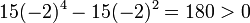$15(-2)^4 - 15(-2)^2 = 180 > 0$, so by the one-sided derivative test, we obtain an endpoint minimum at -2.
• The left-hand derivative at 3 is$15(3)^4 - 15(3)^2 = 1080 > 0$, so by the one-sided derivative test, we obtain an endpoint maximum at 3.

We can also compute the local and endpoint extreme values:

• The value at$x = -2$ (endpoint minimum) is$3(-2)^5 - 5(-2)^3 + 7 = -49$.
• The value at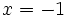$x = -1$ (local maximum) is$3(-1)^5 - 5(-1)^3 + 7 = 9$.
• The value at$x = 1$ (local minimum) is$3(1)^5 - 5(1)^3 + 7 = 5$.
• The value at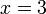$x = 3$ (endpoint maximum) is$3(3)^5 - 5(3)^3 + 7 = 601$.